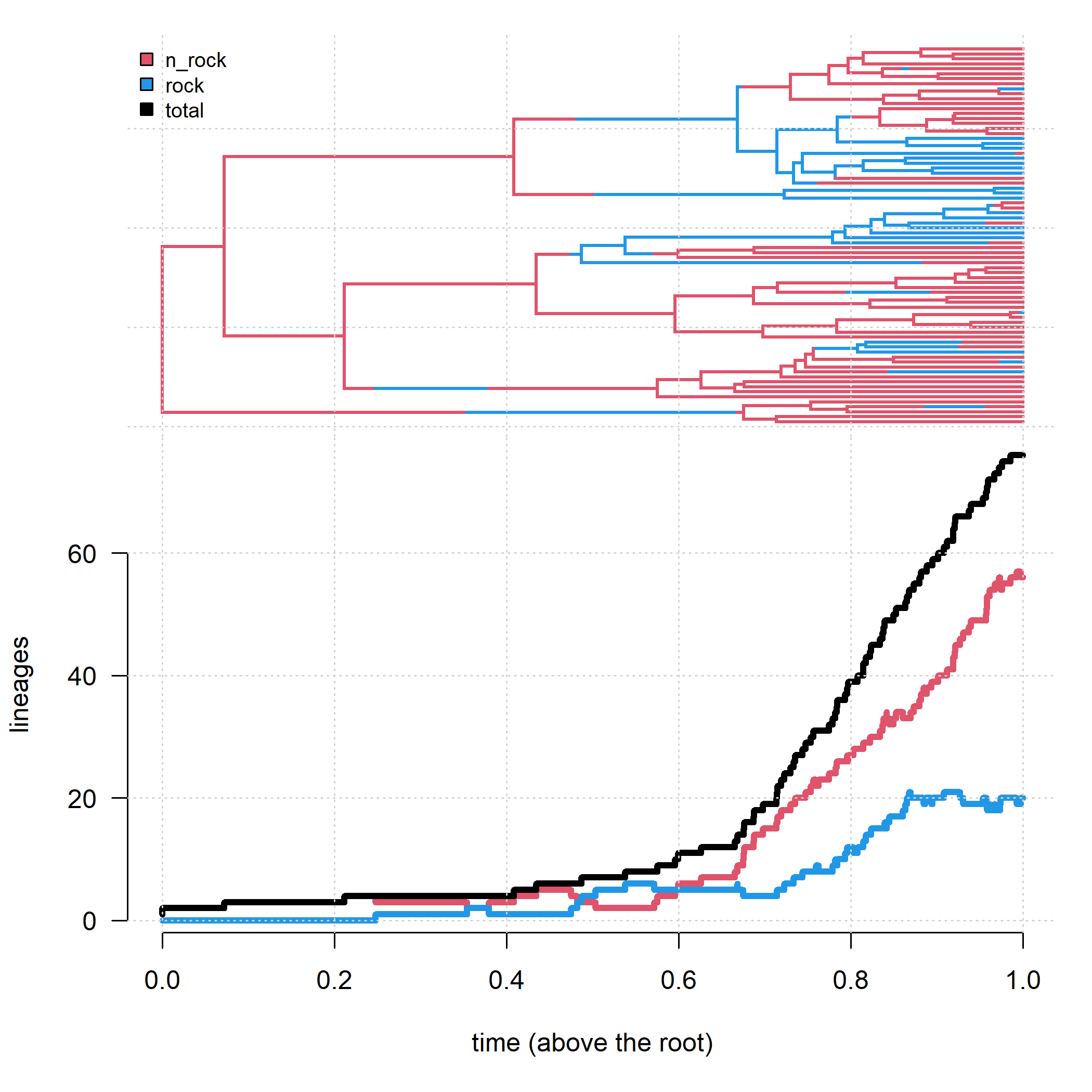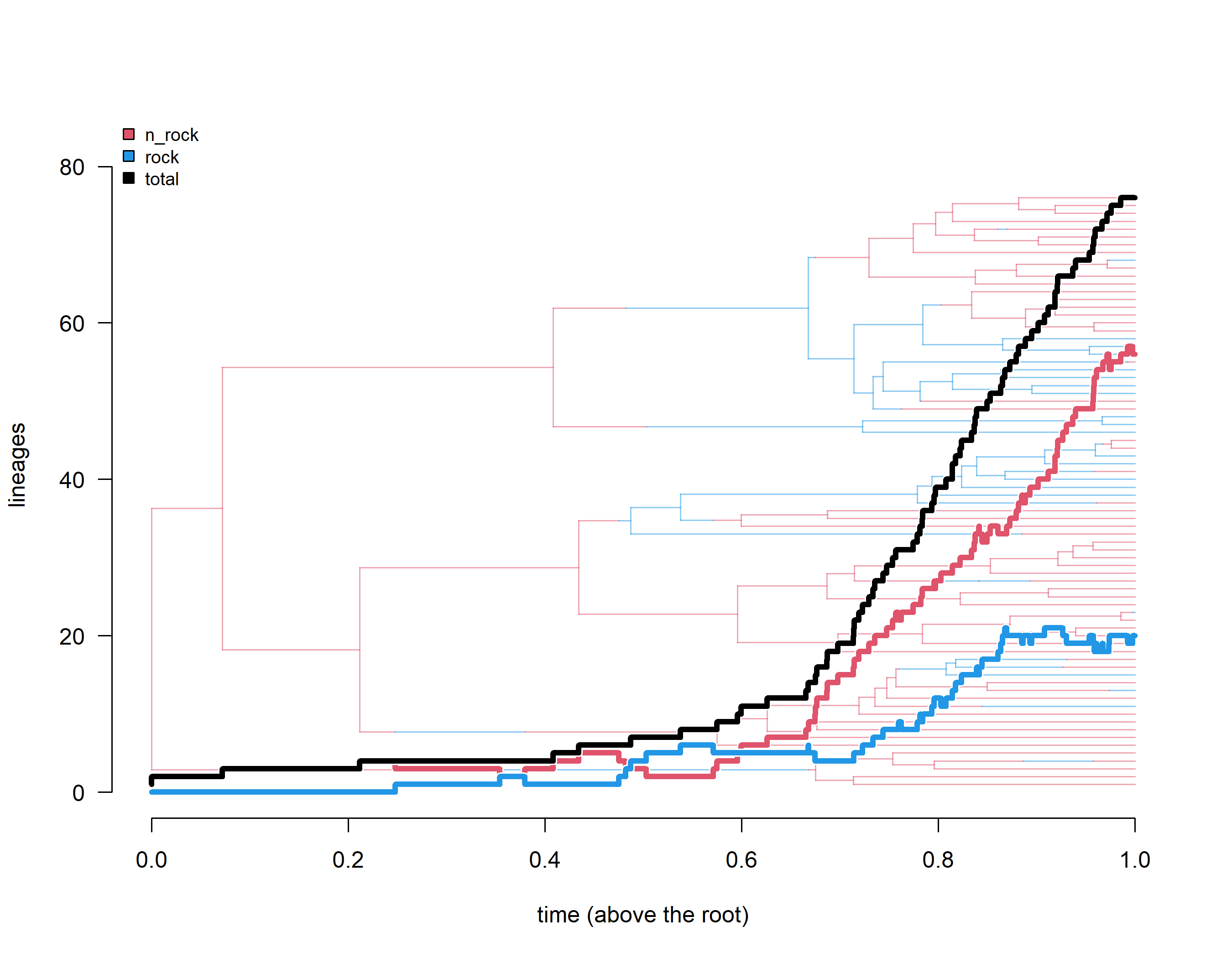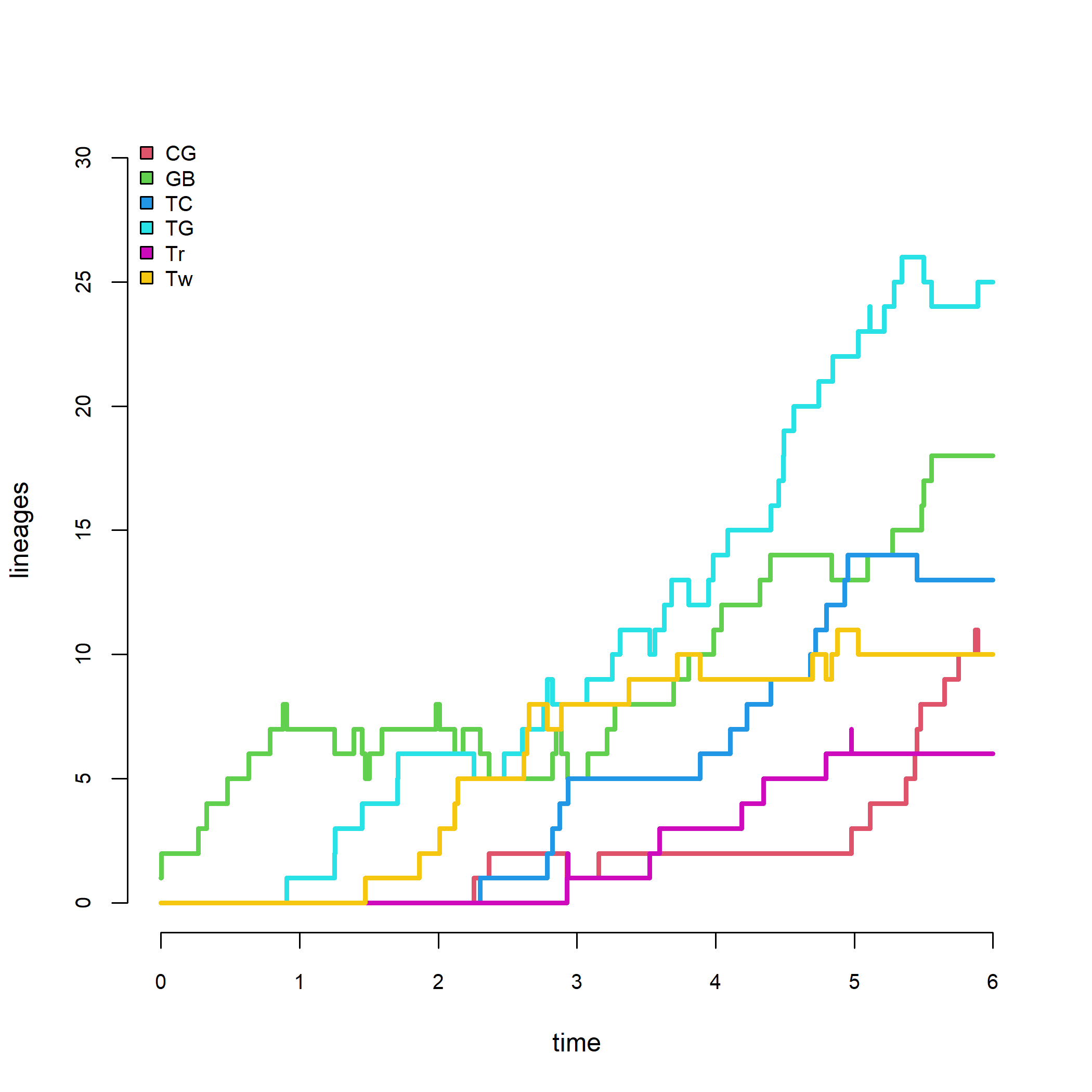## Monday, August 1, 2022

### Lineage-through-time plots for stochastically mapped character histories on the tree now in phytools

A few days ago, I tweeted about creating a lineage-through-time plot from a stochastic character map tree in which we illustrate the accumulation through time of lineages in each of the mapped states.

The tweet blew up more than I expected, so I decide it might be worthwhile to add this as a method to the phytools package.

Rather than proliferate the number of functions of phytools I decided to instead create a new S3 method `ltt`, with functions for different object classes: `"phylo"`, `"multiPhylo"`, `"simmap"`, etc.

This makes it pretty easy to use: we just call `ltt` and then, depending on the object class, R knows which function to use. On the other hand, I also exported `ltt.phylo`, `ltt.multiPhylo`, `ltt.simmap`, etc. to the phytools namespace so that if (for example) we want to call `ltt.phylo` on our `"simmap"` object (this is valid) we can do that too.

To get this new functionally, users will have to update phytools from GitHub, which can be done most easily using the handy devtools package.

``````devtools::install_github("liamrevell/phytools")
``````

To start, here's a demo just reiterating what I showed on my blog a couple of days ago. This is a phylogeny & dataset for rock- and non-rock-dwelling tropidurid lizards that features in an upcoming paper by Ken Toyama, Luke Mahler, & I. The tree has a discrete character encoded, so we're going to pull that out and then run our stochastic mapping, whereas normally we'd start with a phylogeny & discrete character and then do stochastic mapping from there.

``````library(phytools)
url<-"https://raw.githubusercontent.com/liamrevell/evolvcv.lite.figures/main/tropidurid-tree.tre"
habitat<-getStates(lizard.tree,"tips")
habitat<-as.factor(habitat)
lizard.tree<-as.phylo(lizard.tree)
lizard.maps<-make.simmap(lizard.tree,habitat,pi=c(1,0),
model="ARD",nsim=100)
``````
``````## make.simmap is sampling character histories conditioned on
## the transition matrix
##
## Q =
##           n_rock      rock
## n_rock -1.035433  1.035433
## rock    3.460059 -3.460059
## (estimated using likelihood);
## and (mean) root node prior probabilities
## pi =
## n_rock   rock
##      1      0
``````
``````## Done.
``````
``````lizard.maps
``````
``````## 100 phylogenetic trees with mapped discrete characters
``````
``````obj<-ltt(lizard.maps[],plot=FALSE)
obj
``````
``````## Object of class "ltt.simmap" containing:
##
## (1) A phylogenetic tree with 76 tips, 75 internal
##     nodes, and a mapped state with 2 states.
##
## (2) A matrix containing the number of lineages in each
##     state (ltt) and event timings (times) on the tree.
##
## (3) A value for Pybus & Harvey's "gamma" statistic of
##     gamma = -2.4531, p-value = 0.0142.
``````

I've created my `"ltt.simmap"` class object. Now let's plot it!

``````layout(matrix(c(1,2),2,1),heights=c(0.4,0.6))
cols<-setNames(palette()[c(2,4,1)],c(levels(habitat),
"total"))
plot(lizard.maps[],cols,ftype="off",lwd=2,
mar=c(0,4.1,1.1,1.1))
grid()
legend("topleft",names(cols),pch=22,pt.bg=cols,
bty="n",cex=0.8,pt.cex=1.2)
par(mar=c(5.1,4.1,0,1.1))
plot(obj,colors=cols,bty="n",las=1,lwd=4,show.tree=FALSE,
legend=FALSE,ylim=c(1,Ntip(lizard.tree)),
xlab="time (above the root)")
grid()
``````Just as we can for `plot.ltt.phylo`, we're can also superimpose the mapped tree on our lineage through time graph. Let's see that.

``````plot(obj,colors=cols,bty="n",las=1,lwd=4,show.tree=TRUE,
xlab="time (above the root)")
``````Neat.

Finally, for fun, let's try a case with a character that has more than two states.

For this example, I'll load a tree of Anolis lizards. Like the previous example, this tree also contains the discrete state encoded (so we have to pull it out to run our stochastic mapping).

``````data(anoletree)
ecomorph<-as.factor(getStates(anoletree,"tips"))
anole.maps<-make.simmap(as.phylo(anoletree),ecomorph,model="ER",
nsim=100)
``````
``````## make.simmap is sampling character histories conditioned on
## the transition matrix
##
## Q =
##             CG          GB          TC          TG          Tr          Tw
## CG -0.11570697  0.02314139  0.02314139  0.02314139  0.02314139  0.02314139
## GB  0.02314139 -0.11570697  0.02314139  0.02314139  0.02314139  0.02314139
## TC  0.02314139  0.02314139 -0.11570697  0.02314139  0.02314139  0.02314139
## TG  0.02314139  0.02314139  0.02314139 -0.11570697  0.02314139  0.02314139
## Tr  0.02314139  0.02314139  0.02314139  0.02314139 -0.11570697  0.02314139
## Tw  0.02314139  0.02314139  0.02314139  0.02314139  0.02314139 -0.11570697
## (estimated using likelihood);
## and (mean) root node prior probabilities
## pi =
##        CG        GB        TC        TG        Tr        Tw
## 0.1666667 0.1666667 0.1666667 0.1666667 0.1666667 0.1666667
``````
``````## Done.
``````
``````anole.ltt<-ltt(anole.maps[],show.tree=FALSE,bty="n",
cex.axis=0.8,show.total=FALSE,ylim=c(0,30))
``````That's all for now folks.